## Wednesday, February 27, 2019

### (x, why?) Mini: Cilia

(Click on the comic if you can't see the full image.)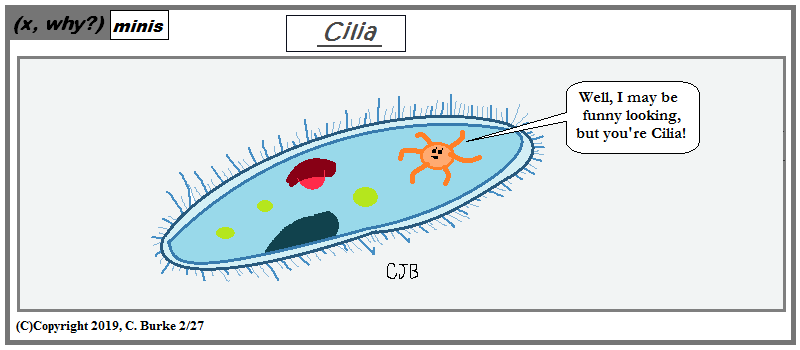Some vacuous science humor.

Come back often for more funny math and geeky comics.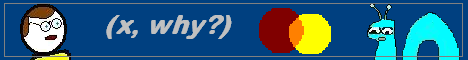## Monday, February 25, 2019

### Angles A,B,C Have Ratio 1:2:3

(Click on the comic if you can't see the full image.)Who doesn't love the occasional class sing-a-long of 'old' songs?

Next problem: find the roots of love. Or your education isn't complete.

Come back often for more funny math and geeky comics.## Friday, February 22, 2019

### Torque

(Click on the comic if you can't see the full image.)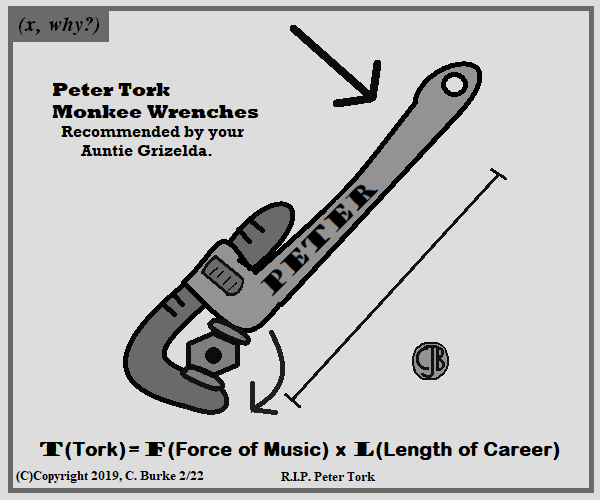What Monkees around, Torks around.
I know I said I'd do fewer obit comics, but The Monkees were the soundtrack to my childhood as much as, if not more than, The Beatles were. (Along with Schoolhouse Rock, which mentioned both groups in the song "A Noun is a Person, Place, or Thing".)

Throwback to January 2009, I did a comic when W was leaving office. Then Ricardo Montalban passed away, and I did a Fantasy Island comic, where I called him "Torque". Despite the comment that I didn't that I didn't know why "I decided to use only shades of gray", no one ever called me on it.

I did the same in this comic as well, for the same reason.

R.I.P. Peter Tork.

Come back often for more funny math and geeky comics.## Thursday, February 21, 2019

### Death Trap Geometry

(Click on the comic if you can't see the full image.)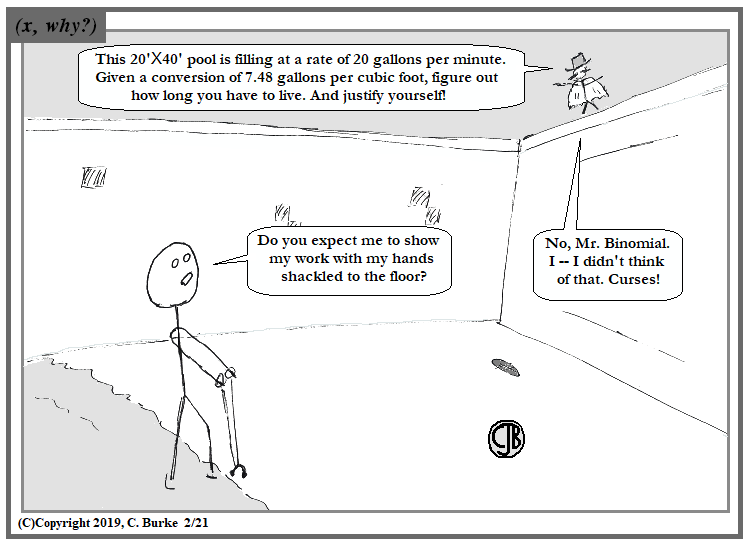Binomial foils again.
Evil VillianTM (a resident of Villeville) was last seen in my old Super-Stick-Man strips, which originated in the 1980s. Yes, his name was originally a spelling mistake that my English teacher gleefully pointed out. I then owned it, explained it, and made that English teacher the psuedo-basis for the character Rotundo.

I was going to go with "Bound" for the agent's name, but "Binomial" is funnier. And the Foil line was serendipitous!

Come back often for more funny math and geeky comics.## Wednesday, February 20, 2019

### January 2019 Common Core Geometry Regents, Part II

The following are some of the multiple questions from the recent January 2019 New York State Common Core Geometry Regents exam.
The answers to Part I can be found here
The answers to Parts III and IV can be found here

### January 2019 Geometry, Part II

Each correct answer is worth up to 2 credits. Partial credit can be given. Work must be shown or explained.

25. Write an equation of the line that is parallel to the line whose equation is 3y + 7 = 2x and passes through the point (2,6).

There are several approaches you can take.
You can rewrite the expression into slope-intercept form, and then solve for "b" using the point (2, 6).

3y + 7 = 2x
3y = 2x - 7
y = 2/3 x - 7/3
y = 2/3 x + b
(6) = 2/3 (2) + b
6 = 4/3 + b
b = 14/3 = 4 2/3
y = 2/3 x + 14/3

You can rewrite the expression into slope-intercept form to get the slope, and then use point-slope form.
(as above, slope is 2/3)
y - 6 = 2/3 (x - 2)

You could replace the 7 with b, and use (2, 6) to find the value that would make the line parallel:

3y + b = 2x
3(6) + b = 2(2)
18 + b = 4
b = -14
3y - 14 = 2x

If you check, you'll see that all three of these are equivalent forms.

26. Parallelogram ABCD is adjacent to rhombus DEFG, as shown below, and FC intersects AGD at H.If m∠B = 118° and m∠AHC = 138°, determine and state m∠GFH.

See image below.
Both figures are parallelograms. Angle B is congruent to angle CDG because opposite angles are congruent, so Angle D = 118.
Angle DGF is congruent to Angle CDG because the alternate interior angles are congruent because the lines are parallel. Angle DGF = 118.
Angle GHF is supplementary to Angle AHC. Supplementary angles have a sum of 180 degrees. 180 - 138 = 42, so Angle GHF = 42.
Triangle FGH has a total of 180 degrees, two of which are 118 and 42, so x + 118 + 42 = 180.
x + 160 = 180, x = 20. Angle GFH = 20 degrees.

27. As shown in the diagram below, secants PWR and PTS are drawn to circle O from external point P.

If m∠RPS = 35°and mRS = 121°, determine and state mWT .

The measure of the angle is one-half of the difference of the two arcs it intercepts.
This means that angle P will be 1/2 (arc RS - arc WT)
Substitute the values and solve for x.
35 = 1/2 (121 - x)
70 = 121 - x
-51 = -x
x = 51 degrees.

28. On the set of axes below, triangle ABC is graphed with coordinates A(-2,-1), B(3,-1), and C(-2,-4). Triangle QRS, the image of triangle ABC, is graphed with coordinates Q(-5,2), R(-5,7), and S(-8,2).

Describe a sequence of transformations that would map triangle ABC onto triangle QRS.

Two methods come to mind:
First, a counterclockwise rotation of 90 degrees about the origin will give you A'(1, -2), etc. Then, reflect over the y-axis, which gives you A"(-1, -2), etc. Finally, a translation to left 4, up 4. T(-4, 4). (There are other combinations of reflection, rotation and translation that will work, depending on the order you do them in.)
Second method: if you reflect over the line y = x, A'B'C' will have the correct orientation, and then will just need a translation. A' will be at (1, -2), and then do T(-4, 4), as in the first method.

29. Given points A, B, and C, use a compass and straightedge to construct point D so that ABCD is a parallelogram.
[Leave all construction marks.]

Construction to be added later. Sorry.
First, the parallelogram is ABCD, so point D must be to the left of point C.
The opposite sides of a parallelogram are congruent.
Starting at point B, open the compass to make an arc through point A. Then move the compass to point C and make an arc to the left side.
Starting at point B, open the compass to make an arc through point C. Then move the compass to point A and make another arc that goes through the first arc you make.
Label the point at the intersection of those two arcs point D. Use the straightedge to make lines CD and AD.

30.
On the set of axes below, triangle DEF has vertices at the coordinates D(1, -1), E(3,4), and F(4,2), and point G has coordinates (3,1). Owen claims the median from point E must pass through point G. Is Owen correct? Explain why.

If EG is a median, then G must be the midpoint of DF. If G is the midpoint, Owen is correct. If it is not, the Owen is not correct. You MUST state one of these.
The x- and y- coordinates of the midpoint must be halfway between those of D and F. You can label this on the graph by counting boxes, or you can use the formula.
To find the middle (the average), add up the two numbers and divide by 2.
x-coordinate: (1 + 5) / 2 = 6 / 2 = 3
y-coordinate: (-1 + 2) / 2 = 1/2
The midpoint is (3, 1/2). Point G is at (3, 1). G is not the midpoint, so the median does not go through G. Owen is not correct.

31. A walking path at a local park is modeled on the grid below where the length of each grid square is 10 feet. The town needs to submit paperwork to pave the walking path. Determine and state, to the nearest square foot, the area of the walking path.

For the two straight pieces, count the boxes and then multiply by 10 * 10 (the scale): 18 * 10 * 10 = 1800.
For the curved pieces: notice that the two semicircles create one complete circle. To find the area of the path, find the area of the bigger circle and subtract the area of the smaller circle.
The larger circle has a radius of 30. The smaller one has a radius of 20.
A = (30)2(pi) - (20)2(pi) = 900(pi) - 400(pi) = 500(pi) = 500(3.141592) = 1570.796
1800 + 1570.796 = 3370.796 = 3371 square feet.

End of Part II

How did you do?

## Tuesday, February 19, 2019

### Stations

(Click on the comic if you can't see the full image.)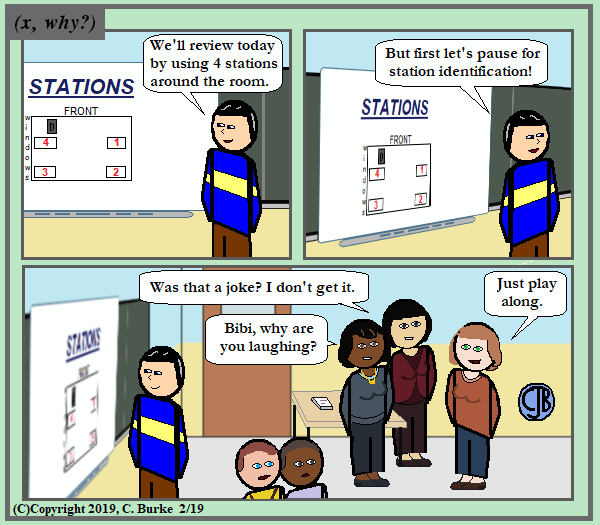Well, it was the top of the class hour.

Come back often for more funny math and geeky comics.## Monday, February 18, 2019

### January 2019 Common Core Algebra 1 Regents, Part I (mult choice)

The following are some of the multiple questions from the recent January 2019 New York State Common Core Algebra I Regents exam.
The answers to Part II can be found here
The answers to Parts III and IV can be found here

Omitted images will be added soon.

### January 2019 Algebra I, Part I

Each correct answer is worth up to 2 credits. No partial credit. Work need not be shown.

1. The scatter plot below shows the relationship between the number of members in a family and the amount of the family's weekly grocery bill.

The most appropriate prediction of the grocery bill for a family that consists of six memebers is

It should be obvious that \$100 is too low and \$500 is too high. \$400 is close to the top end of the range for 7 family members, while \$300 is at the top of the range for 5 family members and in the middle for 7.

2. The function g(x) is defined as g(x) = -2x2 + 3x. The value of g(-3) is

g(-3) = -2(-3)2 + 3(-3)
g(-3) = -2(9) + (-9) = -18 - 9 = -27

3. Which expression results in a rational number?

The square root of 36 is 6. The square root of 225 is 15. The quotient of these numbers is 6/15 or 2/5 or 0.4.
This is a rational number.
In (1), the difference of these two irrational numbers will be irrational. IT WILL NOT BE SQRT(100)!!!!
In (2), the product will be irrational (25 times the square root of 2)
In (4), the sum will be 5 times the square root of 5, which is irrational.

4. The math department needs to buy new textbooks and laptops for the computer science classroom. The textbooks cost \$116.00 each, and the laptops cost \$439.00 each. If the math department has \$6500 to spend and purchases 30 textbooks, how many laptops can they buy?

439x + 116(30) < 6500
439x + 3480 < 6500
439x < 3020
x < 6.87....
They can get 6. Do NOT round up to 7.
You could also have checked each of the choices, multiplying by 439 and adding to 3480, to see which was the largest correct answer.

5. What is the solution to the equation (3/5)(x + 4/3) = 1.04?

(3/5)(x + 4/3) = 1.04
(3/5)x + (3/5)(4/3) = 1.04
(3/5)x + 4/5 = 1.04
3x + 4 = 5.20
3x = 1.2
x = 0.4

I don't like problems that mix decimals and fractions, but what are you going to do?

6. The area of a rectangle is represented by 3x2 - lOx - 8. Which expression can also be used to represent the area of the same rectangle?

Answer: (1) (3x + 2)(x - 4)
Factor the polynomial. (Or multiply the choices.)
The constant term -8 indicates that one binomial has a + and the other has a -.
The middle term is -10. (3)(-4) + (2)(1) = -10, which is Choice (1).

7. Which relation does not represent a function?

In choice (4), each input in the relation is mapped to two outputs. This is not allowed in a function.
In choice (1), the input (x) does not repeat. It is not a linear function, but that isn't what the question asks.
In choice (2), an absolute value function is graphed.
In choice (3), every x has one y value associated with it. (The radical sign means the positive square root, unless preceded by a plus-or-minus symbol.)

8. Britney is solving a quadratic equation. Her first step is shown below.

Problem:3x2 - 8 - lOx = 3(2x + 3) Step 1: 3x2 - lOx - 8 = 6x + 9
Which two properties did Britney use to get to step 1?
III. multiplication property of equality
IV. distributive property of multiplication over addition

The Commutative Property allows rearranging the order of the terms on the left side.
The Distributive Property changed the right side of the equation.

9. The graph of y = (1/2)x2 - x - 4 is shown below.

The points A(-2,0), B(O, -4), and C(4,0) lie on this graph.
Which of these points can determine the zeros of the equation y = (1/2)x2 - x - 4?

Answer: (3) A and C, only
The zeroes are the points where the parabola intersects the x-axis. Point B is the y-intercept.

10. Given the parent function f(x) = x3, the function g(x) = (x - 1)3 - 2 is the result of a shift of f(x)

Answer: (3) 1 unit right and 2 units down
The number inside the parentheses shifts the graph left or right. If there is a minus, the graph moves to the right.
The number outside the parentheses shifts the graph up or down. If it is a minus, the graph moves down.

11. If C = 2a2 - 5 and D = 3 - a, then C - 2D equals

Answer: (3) 2a2 + 2a - 11
Substitution and Distributive property. Then Combine Like Terms.

C - 2D
= (2a2 - 5) - 2(3 - a)
= 2a2 - 5 - 6 + 2a
= 2a2 + 2a - 11

12. Marc bought a new laptop for \$1250. He kept track of the value of the laptop over the next three years, as shown in the table below.

 Years After Purchase Value in Dollars 1 1000 2 800 3 640
Which function can be used to determine the value of the laptop for x years after the purchase?

The laptop is decreasing in value, of choices (1) and (3) are incorrect -- they both show an increase in value. The initial value is \$1250, not \$1000, so choice (2) is incorrect.
You didn't have to calculate the 0.8, but 800/1000 = 0.8 and 640/800 = 0.8, which is the Common Ratio.

13. The height of a ball Doreen tossed into the air can be modeled by the function h(x) = -4.9x2 + 6x + 5, where x is the time elapsed in seconds, and h(x) is the height in meters. The number 5 in the function represents

Answer: (1) the initial height of the ball
When x = 0, h(x) = 5, which is the initial height of the ball.

14. The function f(x) = 2x2 + 6x - 12 has a domain consisting of the integers from -2 to 1, inclusive. Which set represents the corresponding range values for f(x)?

If the domain consists of integers from -2 to 1, then you need to calculate f(-2), f(-1), f(0) and f(1).
You can take a short cut by graphing the function and checking the Table of Values. You'll see that f(-2) = -16, which eliminates choices (1) and (3), and f(0) = -12, which eliminates (4).

15. Which equation has the same solution as x2 + 8x - 33 = O?

Answer: (1) (x + 4)2 = 49
Complete the square.
x2 + 8x - 33 = O
x2 + 8x = 33
x2 + 8x + (8/2)2 = 33 + (8/2)2
x2 + 8x + 16 = 33 + 16
(x + 4)2 = 49

16. The table below shows the weights of Liam's pumpkin, l(w), and Patricia's pumpkin, p(w), over a four-week period where w represents the number of weeks. Liam's pumpkin grows at a constant rate. Patricia's pumpkin grows at a weekly rate of approximately 52%.

Assume the pumpkins continue to grow at these rates through week 13. When comparing the weights of both Liam's and Patricia's pumpkins in week 10 and week 13, which statement is true?

Answer: (3) Liam's pumpkin will weigh more in week 10, and Patricia's pumpkin will weigh more in week 13.
Liam's pumpkin is growing at a rate of 3.1 pounds per week. Over the next four weeks, it will be at 14.8, 17.9, 21.0, and 24.1 in Week 13.
Patricia's pumpkin is growing at a rate of 52%, so each week is 1.52 times the previous week. The next four entries will be (rounded to one decimal place): 13.3, 20.3, 30.8, and 46.9.

17. The function f(x) is graphed below.

The domain of this function is

Choice (1) is eliminated because the graph has a starting point at x = -1. It does not go forever to negative infinity.
If you picked Choice (3), you may have confused domain with range (and x with y).
Choice (2) makes no sense because it says "integers" when rational numbers are included. It also says "positive", even though the graph included the interval [-1, 0].

18. Which pair of equations would have (-1,2) as a solution?

Answer: (3) y = x2 - 3x - 2 and y = 4x + 6
No shortcut. Substitute and check if it makes a true statement.
Choice (1): (2) = (-1) + 2 but (2) =/= 2(-1). 2(-1) = 1/2 or .5
Choice (2): (2) =/= (-1) - 1 even though (2) = 2(1).
Choice (3): (2) = (-1)2 - 3(-1) - 2 = 1 + 3 - 2 = 2. Check.
And 2 = 4(-1) + 6 = -4 + 6 = 2. Check. Correct choice.
Choice (4): 2(-1) + 3(2) = -2 + 6 = 4. Check. 2 = (-1/2)(-1) - 3/2 = 1/2 - 3/2 = -1. Not true.

19. W The formula for electrical power, P, is P = I2R, where I is current and R is resistance. The formula for I in terms of P and R is

There is no common difference, so it is NOT an arithmetic sequence. Choice (1) and (3) out.
Choice (4) makes not sense -- it has a1 in place of the common ratio. If the common ratio was a1, which is 8, that a2 would have to be 8 * 8 = 64, which it is not.
Choice (2) is the only one that makes sense. The common ratio is 20/8 = 2.5. Correct.

20. The formula for electrical power, P, is P = I2R, where I is current and R is resistance. The formula for I in terms of P and R is

Literal equations.
We need to isolate the I, which includes getting rid of the exponent.
Divide by R: I2 = P/R
Square root: I = SQRT(P/R)

21. The functions f(x), q(x), and p(x) are shown below.

When the input is 4, which functions have the same output value?

Answer: (4) f(x), q(x), and p(x)
p(4) = 3, from the table.
f(4) = 3, from the graph.
q(4) = (4 - 1)2 - 6 = 32 - 6 = 9 - 6 = 3
All three functions will equal 3.

22. Using the substitution method, Vito is solving the following system of equations algebraically:

y + 3x = -4
2x - 3y = -21
Which equivalent equation could Vito use?

Answer: (3) 2x - 3(-3x - 4) = -21
If y + 3x = -4, then y = -3x - 4, which can be substituted into the second equation in place of y.
Choices (1) and (2) replaced the x and then changed the y to an x. Crazy talk.
Choice (4) has an arithmetic mistake made when solving for y.

23. W Materials A and B decay over time. The function for the amount of material A is A(t) = l000(0.5)2t and for the amount of material B is B(t) = l000(0.25)t, where t represents time in days. On which day will the amounts of material be equal?

(0.5)2t is the same as ((0.5)2)t, which is the same as (0.25)t.
So A(t) = B(t), and the amounts will always be the same.

24. TThe following conversion was done correctly:

What were the final units for this conversion?

Cross out the common units -- ones found both in the numerator and denominator.
Miles cancels mile
Hour cancels hour
Feet cancels foot.
You are left with inches in the numerator and minutes in the denominator.

End of Part I

How did you do?

## Friday, February 15, 2019

### School Life #8: 4 Eva

(Click on the comic if you can't see the full image.)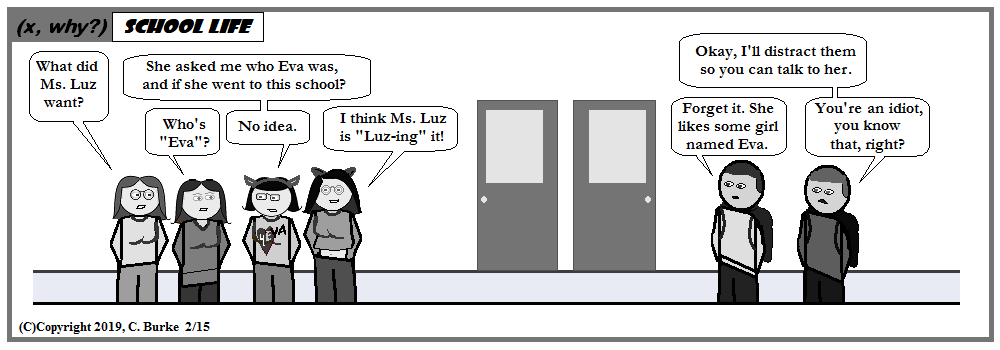There was no way to capture the squeals of delight in the cafeteria yesterday.

I saw this shirt a few days ago, without the accompanying dialogue, however.

Happy Valentines Day!

Come back often for more funny math and geeky comics.## Thursday, February 14, 2019

### I<3U

(Click on the comic if you can't see the full image.)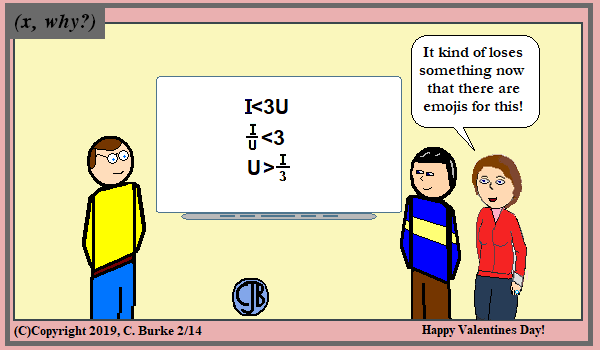Edit:

I may be less than 3 of U, but how do U and I measure up?

Happy Valentines Day!

Come back often for more funny math and geeky comics.## Wednesday, February 13, 2019

### One Line

(Click on the comic if you can't see the full image.)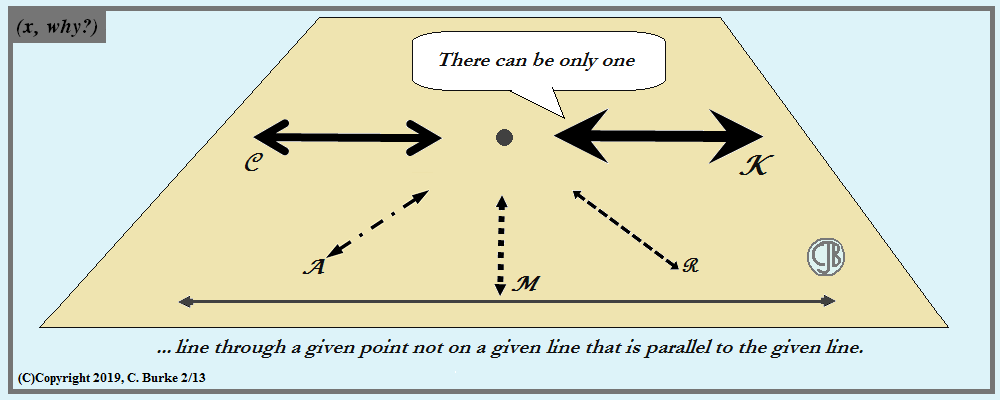Euclider.

I originally planned to have Scotch plains, but the plaid would've been distracting.

Come back often for more funny math and geeky comics.## Monday, February 11, 2019

### (x, why?) Mini: Shell Game

(Click on the comic if you can't see the full image.)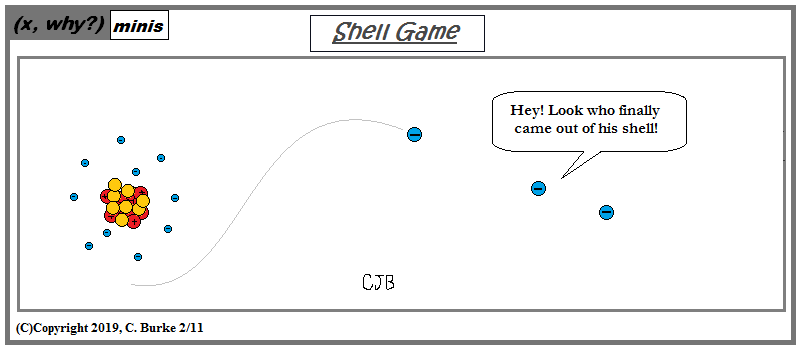Funny or Na?

Now that he's gone the rest of the electrons are a perfect 10!

I know some day soon, I'll regret having used "Shell Game" for a title here. But, hey, I'll just re-use it.

Come back often for more funny math and geeky comics.## Friday, February 08, 2019

### Straight Out of Content

(Click on the comic if you can't see the full image.)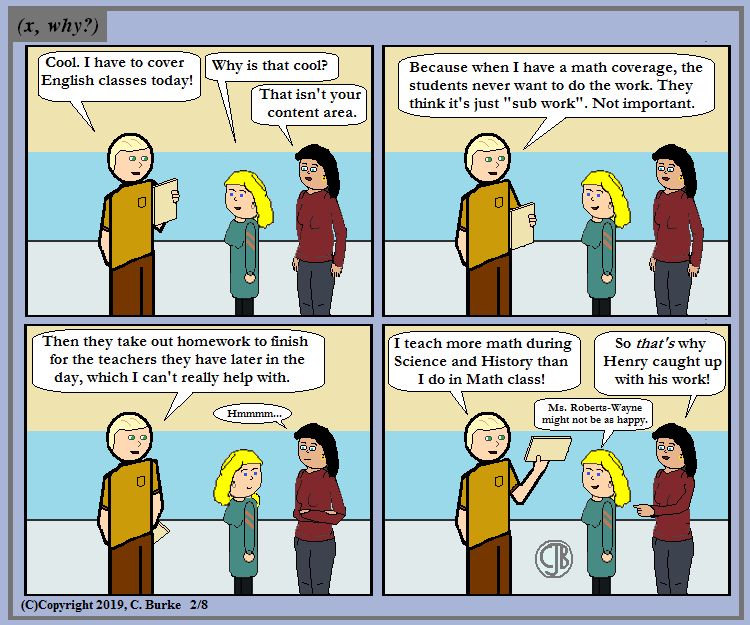Based on a true story -- although once they learn that I actually know math, they usually let teach math in math class.

This was originally title "Subbing Out of Content". And then it wasn't.

Come back often for more funny math and geeky comics.## Tuesday, February 05, 2019

### Engagement and Discussion

(Click on the comic if you can't see the full image.)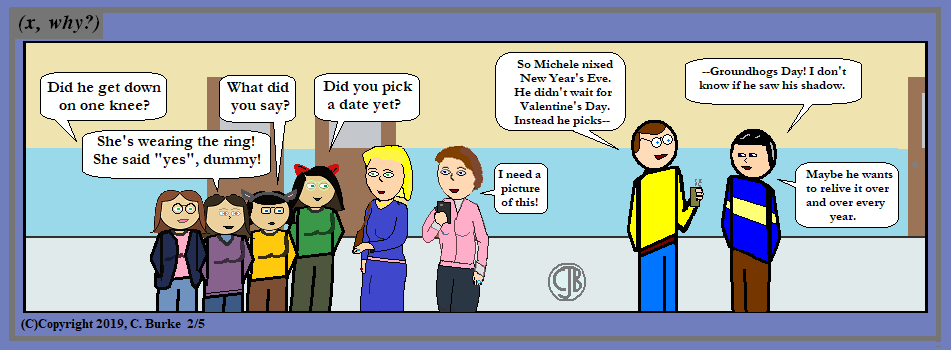Did Chuck predict an early wedding?

Michele prevented Chuck from proposing to Judy on New Year's Eve because that was the night she and Ken got engaged, and she wanted Judy to have her own day. (Or she wanted to her own day to herself?)

In reality, if I don't get around to doing tis, I'll forget it for so long that we'll all just drop the plot line.

Final note: I put some work into the four new girls I made last month. No firm thoughts on what their names might be.

Come back often for more funny math and geeky comics.## Monday, February 04, 2019

### January 2019 Algebra 1 Regents, Parts III & IV

The following are some of the multiple questions from the August 2018 New York State Common Core Algebra I Regents exam.
The answers to Part I can be found here
The answers to Part II can be found here

### August 2018 Algebra I, Part III

Each correct answer is worth up to 4 credits. Partial credit can be given. Work must be shown or explained.

33. Marilyn collects old dolls. She purchases a doll for \$450. Research shows this doll's value will increase by 2.5% each year.
Write an equation that determines the value, V, of the doll t years after purchase.
Assuming the doll's rate of appreciation remains the same, will the doll's value be doubled in 20 years? Justify your reasoning.

2.5% = 0.025, which gets added to 100% = 1.00.
So the equation is V = 450(1.025)t
Substitute t = 20,
V = 450(1.025)20 = 737.377... = \$737.38
\$450 * 2 = \$900, so the doll will not double in value.
Also, (1.025)20 is only 1.638... or 164%, which is less than double (200%).

34. The data given in the table below show some of the results of a study comparing the height of a certain breed of dog, based upon its mass.

Write the linear regression equation for these data, where x is the mass and y is the height. Round all values to the nearest tenth.
State the value of the correlation coefficient to the nearest tenth, and explain what it indicates.

Place all the data into two lists in your graphing calculator and run a linear regression.
You should get a = 1.9 and b= 29.8, so y = 1.9x + 29.8.

Make sure you have DiagnosticsON, or you won't get r, the correlation coefficient.
r = .3, so it is a weak positive correlation.

Use this formula to find the value of V(3) and V(4) and subtract them to find the difference. (You can also graph this in your calculator and check the Table of Values)
V(3) - V(4) = 25000(0.815)4 - 25000(0.815)3
= 13533.584 - 11029.871 = 2503.713 = \$2,503.71 depreciation between years 3 and 4.

35. Myranda received a movie gift card for \$100 to her local theater. Matinee tickets cost \$7.50 each and evening tickets cost \$12.50 each.
If x represents the number of matinee tickets she could purchase, and y represents the number of evening tickets she could purchase, write an inequality that represents all the possible ways Myranda could spend her gift card on movies at the theater.
On the set of axes below, graph this inequality.

What is the maximum number of matinee tickets Myranda could purchase with her gift card? Explain your answer.

13 movies. 13 is the highest whole number on the x-axis lower than the x-intercept. (13, 0) is in the solution set. (14, 0) is not.

36. One spring day, Elroy noted the time of day and the temperature, in degrees Fahrenheit. His findings are stated below.
At 6 a.m., the temperature was 50°F. For the next 4 hours, the temperature rose 3° per hour.
The next 6 hours, it rose 2° per hour.
The temperature then stayed steady until 6 p.m.
For the next 2 hours, the temperature dropped 1° per hour.
The temperature then dropped steadily until the temperature was 56°F at midnight.
On the set of axes below, graph Elroy's data.

State the entire time interval for which the temperature was increasing.
Determine the average rate of change, in degrees per hour, from 6:00 p.m. to midnight.

The temperature is increasing on the graph between 6am and 4pm. (6am, 4pm)
At midnight (12) the temperature is 56. At 6pm, the temperature is 74.
Calculate the rate of change: (56 - 74) / (12 - 6) = -18 / 6 = -3 degrees per hour. The temperature is falling at an average rate of 3 degrees per hour. (If you write it the second way, "falling" implies the negative.)

### August 2018 Algebra I, Part IV

A correct answer is worth up to 6 credits. Partial credit can be given. Work must be shown or explained.

37. A recreation center ordered a total of 15 tricycles and bicycles from a sporting goods store.
The number of wheels for all the tricycles and bicycles totaled 38.
Write a linear system of equations that models this scenario, where t represents the number of tricycles and b represents the number of bicycles ordered.
On the set of axes below, graph this system of equations.
Based on your graph of this scenario, could the recreation center have ordered 10 tricycles? Explain your reasoning.

The equations that you want to graph are

t + b - 15
3t + 2b = 38

You could graph them this way, or rewrite them as
t = 15 - b
t = 38/3 - 2b/3

The slope of the first line is -1. The slope of the second line is -2/3.

You could graph them this way, or rewrite them as
b = 15 - t
b = 38/2 - 3b/2

The slope of the first line is -1. The slope of the second line is -3/2.
The two lines intersect at (8, 7), which is 8 tricycles and 7 bicycles.
Based on the graph, the recreation center could NOT have order 10 tricycles because t=10 is not the point of intersection. (They ordered 8.)

End of Exam

How did you do?

## Friday, February 01, 2019

### Move the Chains!

(Click on the comic if you can't see the full image.)